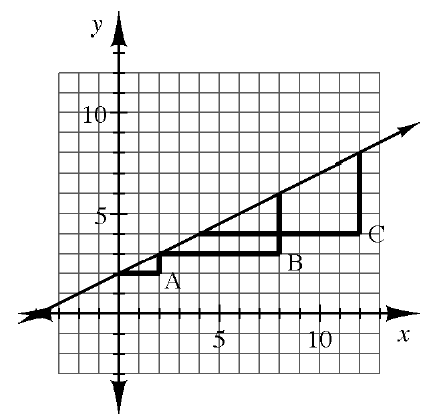### Home > GC > Chapter 4 > Lesson 4.2.3 > Problem4-72

4-72.

Examine the graph at right with slope triangles $\text{A}$, $\text{B}$, and $\text{C}$.

1. Find the slope of the line using slope triangle $\text{A}$, slope triangle $\text{B}$, and then slope triangle $\text{C}$.

Refer to the Math Notes box in Lesson 4.1.1.

2. Hernisha’s slope triangle has a slope of $\frac { 1 } { 2 }$. What do you know about her line?

How does her slope relate to the slope of the line above?